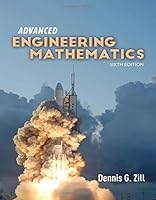# Advanced Engineering Mathematics, 6th Edition## Book Description

Modern and comprehensive, the new sixth edition of award-winning author, Dennis G. Zill's Advanced Engineering is a compendium of topics that are most often covered in courses in engineering , and is extremely flexible to meet the unique needs of courses ranging from ordinary differential equations, to vector calculus, to partial differential equations. A key strength of this best-selling text is the author's emphasis on differential equations as mathematical models, discussing the constructs and pitfalls of each. An accessible writing style and robust pedagogical aids guide students through difficult concepts with thoughtful explanations, clear examples, interesting applications, and contributed problems.

New and Key Features:

• Enhanced - Available with WebAssign Online Homework and Grading System, which includes thousands of new problems for this edition
• NEW - Chapters on differential equations include many new applications and problems
• NEW -Incorporates a new emphasis on integral-defined solutions of differential equations
• Updated - An updated design with new art and photos throughout the text provides an enhanced look and feel
• NEW - Additional Remarks throughout the text provide added clarity to concepts presented in the chapter
• Student Favorite - Includes eight contributed applied project problems spread throughout the text, including an in-depth discussion of the mathematics and history of the Paris Guns of World War I

Every new print copy includes access to the Navigate Student Companion Website where students will find a wealth of learning and study tools to help them succeed in their course, including:

• Projects and Applications contributed by experts in the field
• Two additional chapters on Probability and Statistics

PART 1 Ordinary Differential Equations
Chapter 1 Introduction To Differential Equations
Chapter 2 First-Order Differential Equations
Chapter 3 Higher-Order Differential Equations
Chapter 4 The Laplace Transform
Chapter 5 Series Solutions Of Linear Differential Equations
Chapter 6 Numerical Solutions Of Ordinary Differential Equations
PART 2 Vectors, Matrices, and Vector Calculus
Chapter 7 Vectors
Chapter 8 Matrices
Chapter 9 Vector Calculus
PART 3 of Differential Equations
Chapter 10 Systems Of Linear Differential Equations
Chapter 11 Systems Of Nonlinear Differential Equations
PART 4 Partial Differential Equations
Chapter 12 Orthogonal And Fourier Series
Chapter 13 Boundary-Value Problems In Rectangular Coordinates
Chapter 14 Boundary-Value Problems In Other Coordinate Systems
Chapter 15 Integral Transform Method
Chapter 16 Numerical Solutions Of Partial Differential Equations
PART 5
Chapter 17 Functions Of A Complex Variable
Chapter 18 In The Complex Plane
Chapter 19 Series And Residues
Chapter 20 Conformal Mappings

Appendix I: Derivative and Integral Formulas
Appendix II: Gamma Function
Appendix III: Table of Laplace Transforms
Appendix IV: Conformal Mappings

## Book Details

• Title: Advanced Engineering Mathematics, 6th Edition
• Author:
• Length: 1024 pages
• Edition: 6
• Language: English
• Publisher:
• Publication Date: 2016-09-14
• ISBN-10: 1284105903
• ISBN-13: 9781284105902